Importance: High ✭✭✭
 Author(s): Snevily, Hunter S.
 Subject: Number Theory » Combinatorial Number Theory
 Keywords: addition table latin square transversal
 Recomm. for undergrads: yes
 Posted by: mdevos on: October 13th, 2007
Conjecture   Letbe an abelian group of odd order and let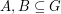satisfy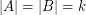. Then the elements ofandmay be orderedand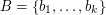so that the sums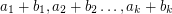are pairwise distinct.

The motivation for this question comes from the study of latin squares. The addition table of every (additive) group forms a latin square, and this gives us a rich source of interesting squares. To explain further, we require a couple of definitions. A transversal of a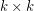matrix is a collection ofcells, no two of which are in the same row or column, and we say that a transversal is latin if no two of its cells contain the same element. Latin transversals are nice structures to find in latin squares. In particular, note that the cells of alatin square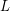may be partitioned intolatin transversals if and only if there is a latin square orthogonal to(see this for a definition of orthogonal latin squares). The above conjecture is perhaps most naturally phrased in terms of latin transversals as follows.

Conjecture  (Snevily's conjecture - version 2)   Everysubmatrix of the addition table of every abelian group of odd order has a latin transversal.

Snevily's conjecture was proved by Alon [A] for abelian groups of prime order using a fairly standard application of the Alon-Tarsi polynomial technique. Later, Dasgupta, Karolyi, Serra, and Szegedy [DKSSz] used a sneaky application of the same technique to prove the conjecture for cyclic groups of odd order (the key to their approach is the fact that forodd,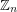is a subgroup of the multiplicative group of the field of order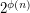whereis Euler's totient function). The conjecture is still open for non-cyclic groups.

The full addition table of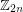does not have a latin transversal. To see this, note that the sum of the elements in this group is equal to(here we identify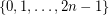within the usual manner). So, if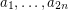andare two orderings of, then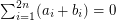, and thereforecannot be an ordering of. This parity problem is the only obstruction known, and the following conjecture asserts that apart from it, the above conjectures holds for cyclic groups of even order.

Conjecture  (Snevily)   Everysubmatrix of the addition table ofhas a latin transversal, unless it is a translate of a cyclic subgroup ofof even order.

In fact, it appears that the above conjecture might hold withreplaced by any abelian group.

## Bibliography

[A] N. Alon, Additive Latin transversals. Israel J. Math. 117 (2000), 125--130. MathSciNet

[DKSSz] S. Dasgupta, Gy. Károlyi, O. Serra, B. Szegedy, Transversals of additive Latin squares. Israel J. Math. 126 (2001), 17--28. MathSciNet

*[S] H. S. Snevily, Unsolved Problems: The Cayley Addition Table of Z. Amer. Math. Monthly 106 (1999), no. 6, 584--585. MathSciNet.

* indicates original appearance(s) of problem.